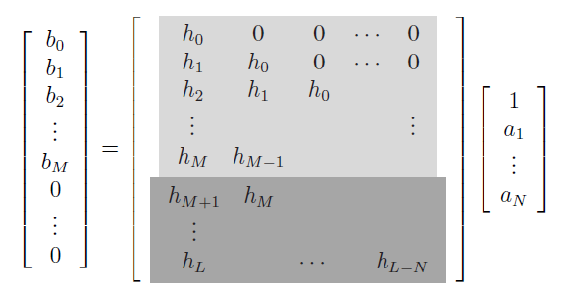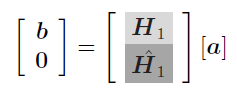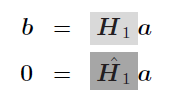# 0.17 Appendix: more on prony and pade

 Page 1 / 1

## Iir filter design using the methods by prony and pade

Consider the filter in [link] with transfer function

$H\left(z\right)=\frac{B\left(z\right)}{A\left(z\right)}$

We can rewrite it as

$B\left(z\right)=H\left(z\right)A\left(z\right)$

which represents the convolution $b\left(n\right)=h\left(n\right)*a\left(n\right)$ . This operation can be represented in matrix form [link] as follows,If $L=M+N$ , equations [link] and [link] describe the same square systems as equations [link] and [link] , letting ${c}_{k}$ in the former systems to become ${h}_{k}$ in the latter ones. Therefore when the number of impulse response samples to be matched is equal to $M+N$ , solving [link] for the coefficients ${a}_{k}$ and ${b}_{k}$ is equivalent to applying Pade's method to matching the first $N+M$ values of the impulse response $h\left(n\right)$ . In consequence, this method is known as Pade's method for IIR filter design [link] or simply Pade approximation method [link] , [link] . Pade's method is an interpolation algorithm; since the number of samples to be interpolated must be equal to the number of filter parameters, Pade's method is limited to very large filters, which makes it impractical.

The relationship of the above method with Prony's method can be seen by posing Prony's method in the $Z$ -domain. Consider the function $f\left(n\right)$ from [link] ,

$f\left(n\right)=\sum _{k=1}^{N}{c}_{k}{e}^{{s}_{k}n}=\sum _{k=1}^{N}{c}_{k}{\lambda }_{k}^{n}$

The $Z$ -transform of $f\left(n\right)$ is given by

$F\left(z\right)=\mathcal{Z}\left\{f\left(n\right)\right\}=\sum _{n=-\infty }^{\infty }f\left(n\right){z}^{-n}$

where $\mathcal{Z}\left\{·\right\}$ denotes the $Z$ -transform operator. By linearity of the $Z$ -transform [link] ,

$F\left(z\right)=\mathcal{Z}\left\{f\left(n\right)\right\}=\sum _{k=1}^{N}{c}_{k}\phantom{\rule{0.277778em}{0ex}}\mathcal{Z}\left\{{\lambda }_{k}^{n}\right\}$

Using

$\mathcal{Z}\left\{{\lambda }_{k}^{n}\right\}=\mathcal{Z}\left\{{\lambda }_{k}^{n}u\left(n\right)\right\}=\frac{1}{1-{\lambda }_{k}{z}^{-1}}$

(the left equality is true since $f\left(n\right)=0$ for $n<0$ by assumption) in [link] we get

$\begin{array}{ccc}\hfill F\left(z\right)& =& \sum _{k=1}^{N}\frac{{c}_{k}}{1-{\lambda }_{k}{z}^{-1}}\hfill \\ & =& \frac{{c}_{1}}{1-{\lambda }_{1}{z}^{-1}}+\frac{{c}_{2}}{1-{\lambda }_{2}{z}^{-1}}+\cdots \frac{{c}_{N}}{1-{\lambda }_{N}{z}^{-1}}\hfill \\ & =& \frac{{c}_{1}\left(1-{\lambda }_{2}{z}^{-1}\right)\cdots \left(1-{\lambda }_{N}{z}^{-1}\right)+\cdots {c}_{N}\left(1-{\lambda }_{1}{z}^{-1}\right)\cdots \left(1-{\lambda }_{N-1}{z}^{-1}\right)}{\left(1-{\lambda }_{1}{z}^{-1}\right)\left(1-{\lambda }_{2}{z}^{-1}\right)\cdots \left(1-{\lambda }_{N}{z}^{-1}\right)}\hfill \\ & =& \frac{\sum _{i=1}^{N}{c}_{i}\prod _{\begin{array}{c}j=1\\ j\ne i\end{array}}^{N}\left(1-{\lambda }_{j}{z}^{-1}\right)}{\prod _{k=1}^{N}\left(1-{\lambda }_{k}{z}^{-1}\right)}\hfill \end{array}$

The numerator and denominator in [link] are two polynomials in ${z}^{-1}$ of degrees $N-1$ and $N$ respectively. Expanding both polynomials, equation [link] becomes

$F\left(z\right)=\frac{{b}_{0}+{b}_{1}{z}^{-1}+\cdots +{b}_{N-1}{z}^{-\left(N-1\right)}}{1+{a}_{1}{z}^{-1}+\cdots +{a}_{N}{z}^{-N}}$

Assuming ${a}_{0}=1$ does not affect the formulation of $F\left(z\right)$ . This is equivalent to dividing both the numerator and denominator of [link] by ${a}_{0}$ . From [link] it is clear that Prony's method is in fact a particular case of the Pade approximation method described earlier in this section (with $M=N$ ). From [link] and [link] we have

$\frac{{c}_{1}}{1-{\lambda }_{1}{z}^{-1}}+\frac{{c}_{2}}{1-{\lambda }_{2}{z}^{-1}}+\cdots \frac{{c}_{N}}{1-{\lambda }_{N}{z}^{-1}}=\frac{{b}_{0}+{b}_{1}{z}^{-1}+\cdots +{b}_{N-1}{z}^{-\left(N-1\right)}}{1+{a}_{1}{z}^{-1}+\cdots +{a}_{N}{z}^{-N\right)}}$

Therefore, given $2N$ samples of $h\left(n\right)=f\left(n\right)$ one can solve for the parameters ${c}_{k}$ and ${\lambda }_{k}$ in [link] merely by applying partial fraction expansion [link] on [link] .

Consider the systems in [link] and [link] . If $L>M+N$ then [link] is an overdetermined system and cannot be solved exactly in general. However, it is possible to find a least squares approximation following an approach similar to the one used in the frequency domain design method from [link] . Given $L>M+N$ samples of an impulse response $h\left(n\right)$ , we rewrite ${\stackrel{^}{\mathbf{H}}}_{1}$ as

${\stackrel{^}{\mathbf{H}}}_{1}=\left[\begin{array}{cc}\stackrel{^}{h}& {H}_{2}\end{array}\right]$

where

$\begin{array}{ccc}\hfill \stackrel{^}{h}=\left[\begin{array}{c}{h}_{M+1}\\ ⋮\\ {h}_{L}\end{array}\right]& \text{and}& {H}_{2}=\left[\begin{array}{cccc}{h}_{M}& {h}_{M-1}& \cdots & \\ {h}_{M+1}& {h}_{M}& & \\ ⋮& & \ddots & ⋮\\ {h}_{L-1}& & \cdots & {h}_{L-N}\end{array}\right]\hfill \end{array}$

Following the same formulation of [link] , the filter coefficients ${a}_{k}$ and ${b}_{k}$ are found by solving for $\stackrel{^}{a}$ and $b$ in

$\stackrel{^}{a}=-{\left[{H}_{2}^{T}{H}_{2}\right]}^{-1}{H}_{2}^{T}\stackrel{^}{h}$
$b={H}_{1}a$

where ${H}_{1}$ is an $M×N$ matrix given by

${H}_{1}=\left[\begin{array}{ccccc}{h}_{0}& 0& 0& \cdots & 0\\ {h}_{1}& {h}_{0}& 0& \cdots & 0\\ {h}_{2}& {h}_{1}& {h}_{0}& & \\ ⋮& ⋮& & \ddots & ⋮\\ {h}_{M}& {h}_{M-1}& \cdots \end{array}\right]$

The error analysis for these algorithms is equivalent to the one performed in [link] . In fact, both approaches (frequency sampling versus Pade's method) are quite similar. Among the common properties, both methods use an equation error criterion rather than the more useful solution error. However, it is worth to point out that in the time domain (Pade) approach, samples of the impulse response are used to make the approximation, and the method uses a linear convolution rather than the cyclic one from the frequency domain approach. Also, since the latter method uses a uniform sampling grid within the complete frequency spectrum between $\omega =0$ and $\omega =2\pi$ instead of using the first few samples of an infinitely long sequence ( $h\left(n\right)$ ), the approximation properties of the frequency domain method are superior.

show that the set of all natural number form semi group under the composition of addition
explain and give four Example hyperbolic function
_3_2_1
felecia
⅗ ⅔½
felecia
_½+⅔-¾
felecia
The denominator of a certain fraction is 9 more than the numerator. If 6 is added to both terms of the fraction, the value of the fraction becomes 2/3. Find the original fraction. 2. The sum of the least and greatest of 3 consecutive integers is 60. What are the valu
1. x + 6 2 -------------- = _ x + 9 + 6 3 x + 6 3 ----------- x -- (cross multiply) x + 15 2 3(x + 6) = 2(x + 15) 3x + 18 = 2x + 30 (-2x from both) x + 18 = 30 (-18 from both) x = 12 Test: 12 + 6 18 2 -------------- = --- = --- 12 + 9 + 6 27 3
Pawel
2. (x) + (x + 2) = 60 2x + 2 = 60 2x = 58 x = 29 29, 30, & 31
Pawel
ok
Ifeanyi
on number 2 question How did you got 2x +2
Ifeanyi
combine like terms. x + x + 2 is same as 2x + 2
Pawel
x*x=2
felecia
2+2x=
felecia
Mark and Don are planning to sell each of their marble collections at a garage sale. If Don has 1 more than 3 times the number of marbles Mark has, how many does each boy have to sell if the total number of marbles is 113?
Mark = x,. Don = 3x + 1 x + 3x + 1 = 113 4x = 112, x = 28 Mark = 28, Don = 85, 28 + 85 = 113
Pawel
how do I set up the problem?
what is a solution set?
Harshika
find the subring of gaussian integers?
Rofiqul
hello, I am happy to help!
Abdullahi
hi mam
Mark
find the value of 2x=32
divide by 2 on each side of the equal sign to solve for x
corri
X=16
Michael
Want to review on complex number 1.What are complex number 2.How to solve complex number problems.
Beyan
yes i wantt to review
Mark
use the y -intercept and slope to sketch the graph of the equation y=6x
how do we prove the quadratic formular
Darius
hello, if you have a question about Algebra 2. I may be able to help. I am an Algebra 2 Teacher
thank you help me with how to prove the quadratic equation
Seidu
may God blessed u for that. Please I want u to help me in sets.
Opoku
what is math number
4
Trista
x-2y+3z=-3 2x-y+z=7 -x+3y-z=6
can you teacch how to solve that🙏
Mark
Solve for the first variable in one of the equations, then substitute the result into the other equation. Point For: (6111,4111,−411)(6111,4111,-411) Equation Form: x=6111,y=4111,z=−411x=6111,y=4111,z=-411
Brenna
(61/11,41/11,−4/11)
Brenna
x=61/11 y=41/11 z=−4/11 x=61/11 y=41/11 z=-4/11
Brenna
Need help solving this problem (2/7)^-2
x+2y-z=7
Sidiki
what is the coefficient of -4×
-1
Shedrak
A soccer field is a rectangle 130 meters wide and 110 meters long. The coach asks players to run from one corner to the other corner diagonally across. What is that distance, to the nearest tenths place.
Jeannette has $5 and$10 bills in her wallet. The number of fives is three more than six times the number of tens. Let t represent the number of tens. Write an expression for the number of fives.
What is the expressiin for seven less than four times the number of nickels
How do i figure this problem out.
how do you translate this in Algebraic Expressions
why surface tension is zero at critical temperature
Shanjida
I think if critical temperature denote high temperature then a liquid stats boils that time the water stats to evaporate so some moles of h2o to up and due to high temp the bonding break they have low density so it can be a reason
s.
Need to simplify the expresin. 3/7 (x+y)-1/7 (x-1)=
. After 3 months on a diet, Lisa had lost 12% of her original weight. She lost 21 pounds. What was Lisa's original weight?
Got questions? Join the online conversation and get instant answers!

#### Get Jobilize Job Search Mobile App in your pocket Now!By Janet ForresterBy Madison ChristianBy Jonathan LongBy OpenStaxBy OpenStaxBy Anh DaoBy Jazzycazz JacksonBy Stephen VoronBy OpenStaxBy Madison Christian